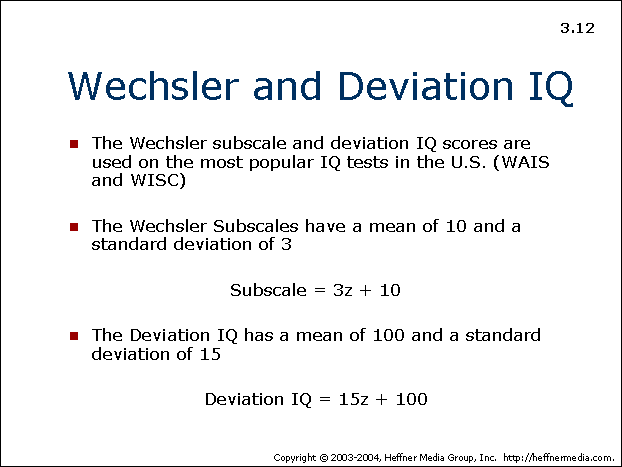# 12: Wechsler and Deviation IQ## Wechsler and Deviation IQ

• The Wechsler subscale and deviation IQ scores are used on the most popular IQ tests in the U.S. (WAIS and WISC)
• The Wechsler Subscales have a mean of 10 and a standard deviation of 3

Subscale = 3z + 10</p align = “center”>

• The Deviation IQ has a mean of 100 and a standard deviation of 15

Deviation IQ = 15z + 100</p align = “center”>